﻿ The Right Speed of Light in a Vacuum (Space) and the Right RelativityPublications are Open
Access in this journal
Article Versions
Export Article
• Normal Style
• MLA Style
• APA Style
• Chicago Style
Research Article
Open Access Peer-reviewed

The Right Speed of Light in a Vacuum (Space) and the Right Relativity

Hany Ali Hussein
International Journal of Physics. 2020, 8(4), 147-152. DOI: 10.12691/ijp-8-4-5
Received October 01, 2020; Revised November 02, 2020; Accepted November 08, 2020

Abstract

This article will propose and empirically validate a new hypothesis for the speed of light. The main result of this proposal is that Galilean/Newtonian relativity is more accurate than special relativity, even for relativistic speeds. The hypothesis is “Light moves in a free space at a speed c, in the inertial reference frame of the smallest celestial particle containing the light source. Once it is released outside the effect zone of this celestial particle, its speed will be c in the inertial reference frame of the bigger celestial particle or the celestial particle that will pick up it. Celestial particles act as carriers for Light.” Another important result for of this proposal is that the absolute/relative speed of light or any other particle may exceed the value c. Through the article, we will deeply validate this proposal using famous phenomena and experiments such as Aberration, Doppler Effect, Binary Stars, Headlight Effect, Michelson-Morley Experiment, and Time Dilation.

1. Introduction

In the 19th century, it was well known and validated that the light speed isn’t impacted with the source speed. However this was not enough to explain some phenomena and experiments such Aberration, Doppler Effect, and Michelson-Morley Experiment. Thus many ideas were proposed.

One of the main ideas was the length contraction due to motion in in the aether. It was introduced by Lorentz. It was complex and obsolete because of no evidence for this idea.

At the beginning of 20th century, another elegant idea was proposed by Einstein. In his theory, Special Relativity, he supposed that the speed of light in a space is constant for all observers, hence introducing the idea of time variance and time space invariance. Gradually, Special Relativity has been accepted in science community. It succeeded to explain all the related phenomena and provided a very close approximate values to the real results of the experiments. That is why it was accepted.

The core idea of special relativity was “introducing the time as a 4th dimension and assuming the space time invariance”. Space-time interval has the same value for all observers. The main concern here is its conflict with the most famous, simple, and logically acceptable relativity; Galilean/Newtonian relativity. In non-relativistic speeds, the results and equations are almost the same. However, in relativistic speeds, there is a difference. Einstein claimed that special relativity results and equations were more accurate, because his theory was the only one able to explain all of these phenomena and experiments.

Now, the question is: can we provide another solution that

• Explain all of these phenomena and experiments?

• Doesn’t contradict with Galilean or Newtonian relativity?

• Provide more accurate results and equations in comparing with special relativity?

If yes, our physics equations and principles will be more consistent and rational. Let us try to answer all of these questions in our proposal. A very important notice is that we don’t try to refute special relativity. We try to propose another solution that should be more accurate and shouldn’t contradict with Galilean/Newtonian relativity. Why not, if this is possible and can be validated?

After Special Relativity, the related works were divided into two main directions:

1. Trials to provide another proposal. But Special Relativity was always the winner. One of the most famous trials, was proposed by Ritz in 1908. He suggested that the speed of light should be c relative to the source. Then he started to explain the light phenomena based on this idea. After five years, this idea was refuted by W. de Sitter who successfully proved that this hypothesis contradicted with the results of binary stars spectroscopic.

2. Experiments to confirm correctness of Special Relativity, such as:

• Ives-Stilwell Experiment performed in 1938, and similar experiments conducted in 1939, 1941, 1962, and 1979 1

• Kennedy-Thorndike Experiment with recent works in 2003, 2004, and 2009 2

• Fast moving clocks with recent works in 2007, 2009, and 2014 3

So, we will focus here on the comparison between our proposal and Special Relativity in some of these phenomena and experiments. In the following sections, we will explain the methodology, discuss the results and experiments used to validate the hypothesis, and conclude the main value of the article.

2. Methodology

Here are, we will use the following experiments and phenomena for validating:

• Aberration

• Doppler Effect

• Michelson-Morley Experiment

• Binary-Stars

• Flight Time and Observed Size of Galaxies

• Fizeau Measurements and Moving Bodies

• Time Dilation.

Before going into details of these methods, I want to highlight some points about the proposal.

If the light source and observer are located on the surface of a planet, such as the Earth, the speed of light in a vacuum is c in the inertial reference frame (IRF) of the Earth. So, if any of them is fixed, the relative speed is c. If for example the observer moves with a speed v relative to the Earth, the relative speed between the light and the observer is c-v. Also, it will have a speed of c + u relative to any observer in our solar system, if the Earth has a speed u relative to this observer. If the light is released outside the effect zone of the Earth (let us say, for simplicity, going outside its atmosphere), the speed of light will be c relative to IRF of our solar system. During the light travel, if it goes into the effect zone of any other planet in our solar system, its speed will be c relative to IRF of this planet.

In this study, we will ignore the acceleration effect of gravitation on photons towards the center of the celestial particle. We will focus only on the displacement effect on the light in the direction of the carrying celestial particle motion.

Free atomic particles, such as electrons or nuclei, have the same effect of celestial particles when the light goes into their effect zone. Now, the question is “what is about emitted photons?” Photons will be emitted with a speed c relative to the electron speed and this continues till going out of its effect zone (may take around 10-19 seconds or less). Then it will move with a speed c relative to the carrying celestial particle.

Regardless the reason of the effect (gravity ….), as mentioned, each celestial or atomic particle has its own effect zone, in which the light will move with a speed c relative to it.

When the light is emitted from a small moving body such as the car lights, we can ignore its effect zone (which nearly equals to the effect zone of the emitting electrons), and consider the light speed is c relative to the celestial particle carrying this body.

Regarding the communications, electromagnetic waves, photons that are interchanged between neighboring atomic particles and responsible for the interconnection between these particles, their speed will be c relative to the emitting atomic particle as long as they are within its effect zone. Then their speed between these particles will be c relative to the carrying celestial particle. When they reach the neighbor atomic particle, again their speed will be c relative to this particle. That is the reason behind the time dilation phenomena. More details are covered in the related section.

Light passing through a moving medium subjects to Fizeau/Fresnel equation. By the end of the article, we will try to correlate between this proposal and Fresnel drag coefficient and conclude one equation.

3. Discussing Experiments and Results

3.1. Aberration
• Figure 1. The apparent position of a star is displaced in the direction of the Earth motion

Relativistic Aberration at right angle is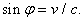(1)

Let us explain Aberration based on this proposal and try to find its angle. When the light reaches the Earth effect zone, it will move with a speed c relative to the Earth. So, both light and observer will move with distance (v/c) L in the motion direction. L is the length of the Earth effect zone (let us say it is equal to the atmosphere length). Then the light hits the telescope at right angle as shown in Figure 2.

• Figure 2. The displacement is L (v/c), where L is the length of the effect zone

The same will happen if the Earth moves in the opposite direction.

So, to determine the mean angle of offset from the right position,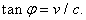(2)

Let us highlight the following points for this equation:

1. It provides almost the same result of the relativistic aberration. However if you seek more accuracy, you will find the results of this equation are closer to the experiments results.

2. It is the same equation of Bradley & Thomas Young, however with a different explanation.

Now let us fill the telescope with water, as performed previously by Francois Arago, Airy & others. Based on Bradley & Young’s assumption, it will be (tan φ = v/(c/nwater). However, the results showed that the angle wasn’t affected by the medium 4. Based on my proposal, it is always (tan φ = v/c), because it is due to the displacement of light when going into the Earth effect zone, not going into the telescope only. So, it is independent of the medium into the telescope.

3. For more accuracy, the displacement depends on travelling time of light through the effect zone (let us say it is equal to atmosphere length (around 500K)) plus the travelling time through the telescope (its length ratio to the total length is too small (0.000002) and can be ignored). So, regardless the medium in the telescope, tan φ always equals to v/c.

4. Part of the effect zone includes air. So, nair should be considered in calculation. If this is done, the results will be closer to the experiments results.

3.2. Doppler Effect

Relativistic Doppler Effect is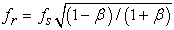(3)

Where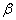=v/c.

Doppler Effect based on this proposal is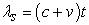(4)

Where v is the relative speed of receiver to the source, and it is in the opposite direction of light propagation. So,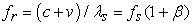(5)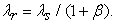(6)

Using readings of Ives-Stilwell experiment done in 1938, we can find that redshift and blue shift Results of this formula are closer to the experiment readings than special relativity’s results.

Please note that in the redshift calculations of this experiment, the equation will be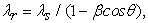(7)

Where θ = 7 degrees, it was the angle at which the backward light was measured 6

Even the difference between readings and formula results is expected. The formula calculates the frequency and wavelength of the exact horizontal light beam. However, not all the light beams of the measured light spot are horizontal. Some of them are deviated upper or lower the horizontal line. The impact of the source speed will be v cos φ for such beams. So, the measured receiver blue shift frequency will be less than the theoretical result, and the measured received blue shift wavelength will be higher than the theoretical result.

• Figure 3. The frequency and wavelength of non-horizontal beams are impacted with their angle with the velocity direction
3.3. Michelson-Morley Experiment

It was performed in 1887 7. Then it was conducted many times. The recent works of the experiment (from 2003 to 2009), used the optical resonator to provide more accuracy. 8, 9

Here, we will show how easily and reasonably explain this experiment.

In this experiment, the Earth is the celestial particle. So, the speed of light is c relative to it. Also, all mirrors are fixed (Zero relative speed to the Earth). So, the speed of light is c relative to the Earth, and relative to all mirrors in the forward and backward directions. Consequently, both longitudinal and transverse time are equal to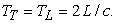(8)
• Figure 4. Simple diagram for Michelson-Morley Experiment

As shown, no need for the artificial of length contraction or space-time invariance. Using this proposal, you can explain it easily using Galilean/Newtonian relativity. Light speed here is c for all observers, because all observers are fixed in IRF of the Earth. However we can’t generalize this rule as Einstein tried. With the same concept, you can easily explain similar experiments such as Kennedy-Thorndike experiment. The travel time of the light beam in any arm will not change if the Earth speed changes.

3.4. Binary Stars

Willem de Sitter used this experiment to easily refute Ritz’s explanation. Actually, he was right. Moreover the results confirmed his argument 10. Using this proposal, each beam light has a speed c relative to star as long as it is within its effect zone. Once they are released, both beams have a speed c relative to the bigger IRF (Galaxy). Both of them will move with a speed c till reaching the receiver. So, as long as the effect zone length of these stars is too much less than the distance between the binary system and the observer, the two beams reach the observer at the same time. If you know, the nearest binary stars to the Earth is about 4 light years distance, i.e. the difference time between the two beams is less than 5 Nano seconds. It is almost equal to 5 parts of million in comparing with the maximum difference time due to different positions of the stars in the orbital.

3.5. Flight Time & Observed Size of Galaxies

This is a good challenge to invalidate this proposal. In all flight time experiments, the moving source and observer were into the same celestial particle (the Earth). So, the speed of light in vacuum was always c in IRF of the Earth, regardless the speed of the source. Let us now imagine another scenario, where the source of light and the observer are in two different celestial particles, and the two particles have a relative speed v. The situation will be different. For example, if another galaxy approaches Milky Way with a speed v, the light, emitted by stars at the front side of this Galaxy, will leave the Galaxy quickly and will have a speed c relative to Milky Way. However the light emitted by stars at the rear side has a speed c relative to their galaxy during the travelling within it (i.e. it has a speed c+ v relative to Milky Way). Then it will have a speed c when leaving its Galaxy. So, for a time, this beam has a speed higher than the first beam. So, if the distance of Stars is calculated based on the travel time of light on old bases (fixed speed c), this star will be closer than it looks as shown in Figure 5. Also, the rear side of this Galaxy will be narrower than the front side. The vice is right for a galaxy moves away from our galaxy as shown in Figure 6.

• Figure 5. The real and apparent positions of a star at the rear side of a Galaxy approaching Milky Way
• Figure 6. The real and apparent positions of a star at the rear side of a Galaxy moving away from Milky Way

The same scenario can be applied for any planet of our solar system. Based on old flight time calculations, its apparent position is nearer than the real position when it approaches the Earth, and the vice in the opposite direction.

As mentioned before, when a celestial particle catches a light beam, it acts as a carrier. This causes a displacement for the light beam or even changing in its direction. But what will happen if the particle emits light? Let us say about a star moving in X-direction in IRF of our Galaxy. In this case, the particle will try to add its velocity to the emission velocity as in Figure 7. However the maximum value is c relative to this particle. So, both X & Y -components will be decreased as shown in Figure 8 to keep the emission speed at value c. The remaining energy will be transferred back to the emitting atoms.

• Figure 7. When a moving source tries to emit a photon at angle θ to the direction of motion, it tries to add its velocity to the emission velocity
• Figure 8. Speed of the photon will be forced to be c, and only the angle of light beam will be impacted by the source speed

The new angle of the beam will be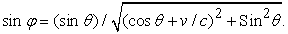(9)

This is the second part of my hypothesis. It briefly explains how the light is emitted.

When θ is 90°,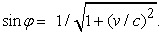(10)

The relativistic beaming, at θ equals 90°, is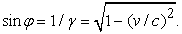(11)

Both angles are inversely proportional to (v/c) 2, with a little bit difference in values. Actually I don’t have enough data to show which one is more accurate.

Another important difference is that, in the relativistic beaming, v is the relative speed between the source and the observer, however in this hypothesis, v is the speed of the source in relative to IRF of the carrying celestial particle. It means that this is a property of emitting particle when moving, regardless the status of the observer. On the other hand, there is another effect when the observer moves towards the source. In this case the intensity of the intercepted light increases gradually. This effect depends on the relative speed between the observer and the source.

Some people see it is more convenient if such phenomena depends on the relative speed, not the independent speed of the source or observer. But I don’t think so. If you remember, the aberration phenomenon depends on the observer motion, and the light-time correction depends on the source motion. So, what is the issue if the headlight depends on the source motion only?

Note: some researchers use the famous equation of relativistic aberration for headlight (relativistic beaming), however this is not right. Equation (11) is the correct one.

3.7. Fizeau Measurements & Moving Bodies

The light moves with:

1. a speed c in the free space relative to the carrying celestial particle

2. a speed c relative to the free atomic particle when goes into its effect zone

3. a speed c/n when moving into a fixed medium. This speed is relative to the celestial particle carrying this medium.

You may ask why in case-3, it doesn’t move with a speed c as happening in case-2. This is an important question, and I’ll answer it in another article. But currently we can briefly say that case-2 is for one free atomic particle. In case-3, the situation is different. The light will pass through the molecular structure of the medium. This will reduce its global speed to c/n relative to this fixed medium or to its carrying celestial particle.

When this medium moves with a speed v, the light moves in the free space between the atomic particles with a speed c/n and with a speed c/n + v when goes into the effect zone of these atomic particles. All of these speed are relative to IRF of the carrying celestial particle. The net speed is between c/n and c/n + v. We can’t predict the exact net speed, it has to be measured. If you go to Fizeau experiment and Fresnel drag coefficient, you will find the net speed 11. It is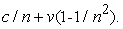(12)

Moving body is the case number 4. Now, can we generate a new form that represent all of these cases? Equation (12) shows the speed of light in IRF of the celestial particle carrying the moving body. So, the speed, in IRF of the moving body, will be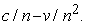(13)

After adding a new constant P, the general form will be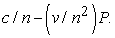(14)

This is the speed of light relative to the moving body. P equals zero, when the moving body is a celestial or free atomic particle. P equals to 1, when the moving body is any other moving medium.

3.8. Time Dilation

Time dilation is due to the delay in communication between the atomic particles. As per special relativity, time dilation factor is: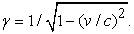(15)

As per this proposal, time dilation factor should be slightly less than this value.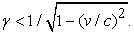(16)

Why this difference? In special relativity, the speed of light or electromagnetic waves between the atomic components is c, independent of the speed of the moving body or its components. However in this proposal, the speed is c relative to the atomic particle as long as it is within the effect zone of this particle. Then it will be c relative to the carrying celestial particle (i.e. it is independent of the atomic particle speed). Then when it reaches the neighbor atomic particle, its speed will be c relative to it. So, the total time dilation should be slightly less than what is predicted by special relativity.

If you check the results of muons lifetimes experiments, you will find that the measured time dilation is less than the expected value of special relativity 12, 13, as per our expectation.

Now and before going to the conclusion, what are the factors that impact special relativity accuracy?

1. When the distance between carrying particles is not big enough in comparing with the effect zone length of these particles.

2. When the relative speed of the moving body to the carrying celestial particle increases. Are you sure? Yes, if the relativistic speed increases, the accuracy of special relativity will decrease.

3. When the light motion is longitudinal to the particles motion, the error increases in comparing with the transverse motion. In longitudinal motion γ should be less than 1/(1-(v/c)2).

In all of these cases, our proposal, in conjunction with Galilean relativity, provides more accuracy.

Note 1: Don’t confuse between the communication between neighboring atomic particles and light passing through a medium in which atomic particles will obstruct its motion and reduce it to c/n.

Note 2: the gravitational time dilation is already implied in the hypothesis definition. Actually, the effect zone of celestial particle is due to its gravitation. So, the gravitation will cause a displacement for photons in its motion direction, and will try to attract these photons to the particle center, as well.

Note 3: in Michelson-Morley Experiment, no any time dilation happens, because all of the source, the receiver, and the light are displaced in the direction of the Earth motion, and the source is a small body that almost doesn’t have an effect zone, as mentioned in page 2.

4. Conclusion

Finally, Light and Electromagnetic waves propagate in a free space at a speed c, in the inertial reference frame of the celestial particle carrying these waves. Consequently, the absolute/relative speed of light in a free space or even any other particles may be higher than c. In addition, Galilean/Newtonian relativity is more accurate than special relativity. Here are, you will find a brief comparison between special relativity and this hypothesis.

Acknowledgements

My wife greatly supported me during this work. Also, Dr. David Halliday helped me to drop my older proposal because it was wrong. Then I came up with this final idea.

References

  D. Hasselkamp, E. Mondry, A. Scharmann, "Direct observation of the transversal Doppler-shift". Zeitschrift für Physik A. 289 (2): 151-155, 1979. In article View Article  M. E. Tobar, P. Wolf, S. Bize, G. Santarelli, V. Flambaum, "Testing local Lorentz and position invariance and variation of fundamental constants by searching the derivative of the comparison frequency between a cryogenic sapphire oscillator and hydrogen maser". Physical Review D. 81 (2): 022003. In article View Article  B. Botermann, D. Bing, C. Geppert, G. Gwinner, T. W. Hänsch, G. Huber, S. Karpuk, A. Krieger, T. Kühl, W. Nörtershäuser, C. Novotny, S. Reinhardt, R. Sánchez, D. Schwalm, T. Stöhlker, A. Wolf, G. Saathoff, Guido, "Test of Time Dilation Using Stored Li+ Ions as Clocks at Relativistic Speed". Physical Review Letters. 113 (12): 120405. In article View Article  PubMed  E.T. Whittaker, "A History of the theories of aether and electricity,” Longmans, Green, London, pp. 120-121, 1910. In article  H. E. Ives, G. R. Stilwell, "An experimental study of the rate of a moving atomic clock". Journal of the Optical Society of America. 28 (7): 215. In article View Article  A.A. Faraj, "The Ives-Stilwell Experiment,” The General Science Journal, pp.2, 2016. In article  A.A. Michelson, E.W. Morley, "On the Relative Motion of the Earth and the Luminiferous Ether" American Journal of Science. 34(203): 333-345. In article View Article  H. Müller, P.L. Stanwix, M.E. Tobar, E. Ivanov, P. Wolf, S. Herrmann, A. Senger, E. Kovalchuk, A. Peters, "Relativity tests by complementary rotating Michelson-Morley experiments". Phys. Rev. Lett. 99(5): 050401. In article View Article  PubMed  Herrmann, S.; Senger, A.; Möhle, K.; Nagel, M.; Kovalchuk, E. V.; Peters, A. (2009). "Rotating optical cavity experiment testing Lorentz invariance at the 10-17 level". Physical Review D. 80 (100): 105011. In article View Article  W. de Sitter, "A proof of the constancy of the velocity of light," Royal Netherlands Academy of Arts and Sciences Proceedings, vol.15, Issue.2, pp.1297-1298, 1913. In article  M.H. Fizeau, "On the Effect of the Motion of a Body upon the Velocity with which it is traversed by Light," Philosophical Magazine, vol.19, Issue.4, pp.245-260, 1860. In article View Article  J. Bailey, K. Borer, F. Combley, H. Drumm, F. Krienen, F. Lange, E. Picasso, W. von Ruden, F. J. M. Farley, J. H. Field, W. Flegel, P.M. Hattersley, “Measurements of relativistic time dilatation for positive and negative muons in a circular orbit,” Nature, 268, pp.301-305, 1977. In article View Article  J. Bailey, K. Borer, F. Combley, H. Drumm, C. Eck, F.J.M. Farley, J.H. Field, W. Flegel, P.M. Hattersley, F. Krienen, F. Lange, G. Lebée, E.Mc Millan, G. Petrucci, E. Picasso, O. Rúnolfsson, W.von Rüden, R.W. Williams, S.Wojcicki1, “Final report on the CERN muon storage ring including the anomalous magnetic moment and the electric dipole moment of the muon, and a direct test of relativistic time dilation,” Nuclear Physics B, vol.150, pp.1-75, 1979. In article View ArticleThis work is licensed under a Creative Commons Attribution 4.0 International License. To view a copy of this license, visit http://creativecommons.org/licenses/by/4.0/